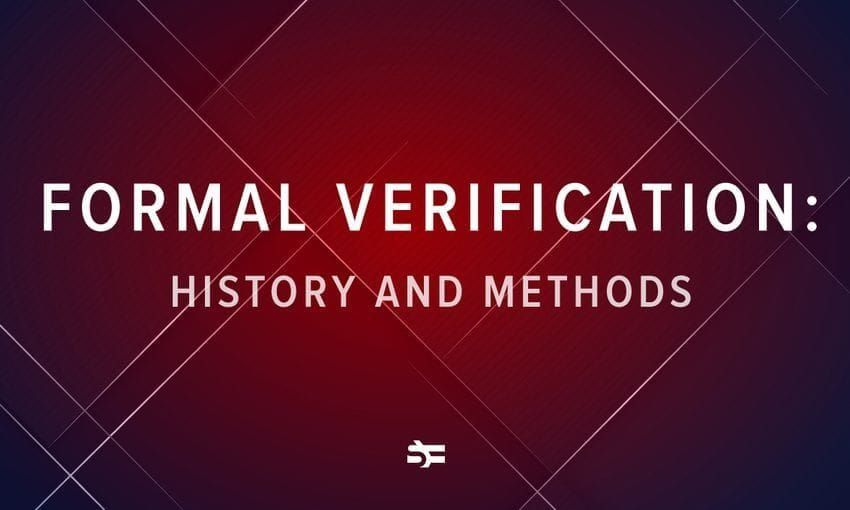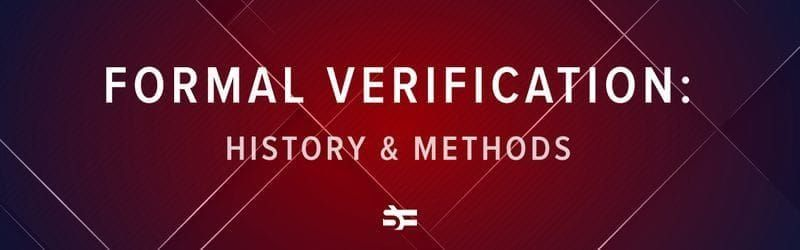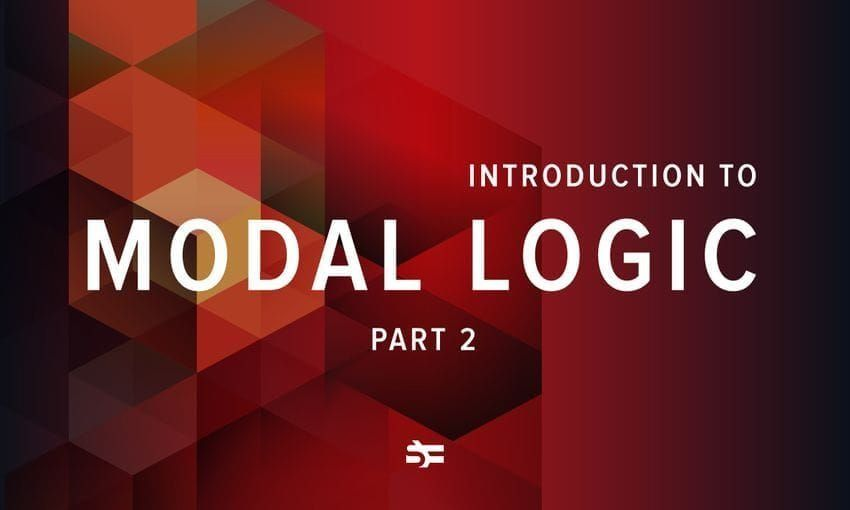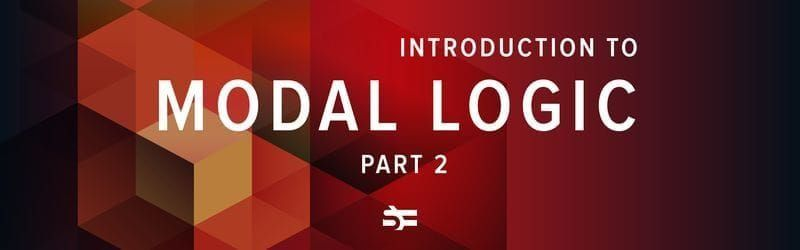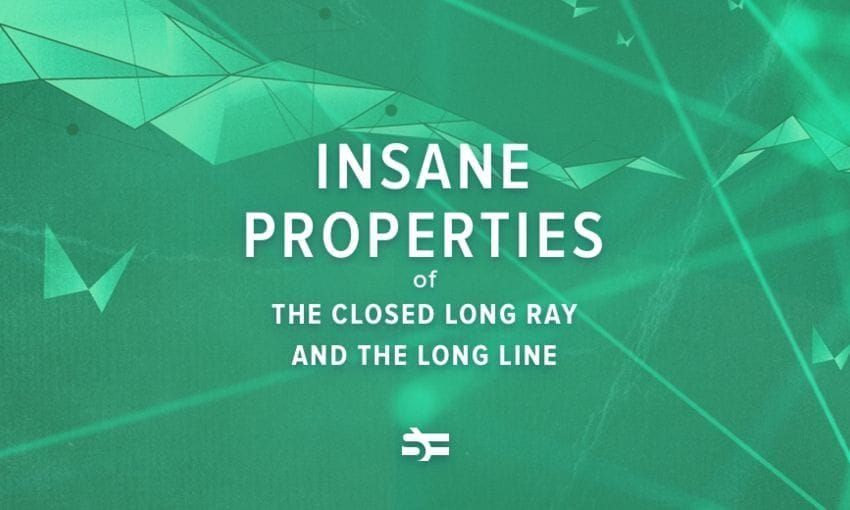# The Greatest Challenge of Modeling Large Quantum Computers

### This article opens a series of guest posts written by our friends from the ‘Mind vs Trash’ VK-community. Young mathematicians, physicists, computer scientists will share their thoughts about cutting-edge topics. Today’s subject is the problem of modeling large quantum computers.

###### Perhaps you cannot quite picture how quantum computers work, but you definitely heard something about them. Nowadays, all rich, as well as not-so-rich states and corporations, are trying to build one. However, many face a problem of inability to emulate a large quantum computer. Let us figure out why.

A traditional computer processes widely-known bits. Here everything is simple: N bits imply N binary cells which at any given time can be either 0 or 1. Quantum computers use quantum bits, i.e., qubits. A qubit state is not binary. The general quantum state of a qubit can be represented by a linear superposition of its two basis vectors which refer to two classical states of a classical bit. However, when changing a qubit value, one of two classical values comes out, but now the qubit has a probability of the value ‘0’ and a probability of the value ‘1’. The mentioned probability is calculated from the vector coordinates.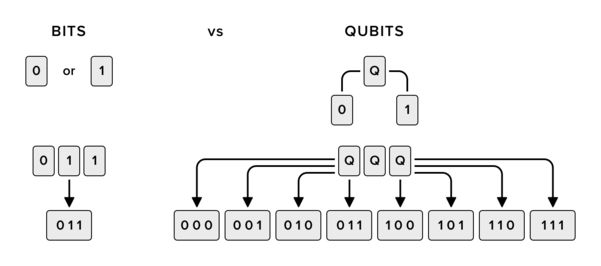It is more or less simple so far but, if we deal with a lot of qubits, it becomes more terrific. The thing is, in quantum mechanics, a tensor product of the spaces of the subsystems refers to the joint states of the two systems. But a tensor product implies that dimensions of the spaces are multiplied. Thus, to describe a 3-qubit system, we need 2^3=8-dimensional space. When we add 1 bit in a traditional computer, the size of the required memory increases by 1 bit, i.e., by 1 binary cell. When emulating a quantum computer using a traditional one, adding 1 qubit results in doubling of the memory in use. Can you see the problem?

For instance, when modeling coordinates of a complex vector of double-precision floating point types which require 8 bytes each, one complex coordinate will require 16 bytes, and N qubits require 16⋅2^N = 2^(N+4) bytes. If your computer memory equals to 8 GB (8⋅2^30 = 2^33 bytes), you’re able to emulate a quantum computer with no more than 29 qubits. I suppose, now the news about a 50-qubit quantum computer sounds more impressive, right?More from Serokell